WEBINAR
External Rotor PMSM Technology for Energy Generation and Durability
Thursday, March 30, 2023
Time
SESSION 1
SESSION 2
CEST (GMT +2)
03:00 PM
08:00 PM
EDT (GMT -4)
09:00 AM
02:00 PM
HOME / Applications / 2D Simulation of a Magnetic Levitation System Used in Semi-High-Speed Maglev Train

# 2D Simulation of a Magnetic Levitation System Used in Semi-High-Speed Maglev Train

Used Tools:## Introduction

Magnetic levitation is a technique whereby an object is hung in the air solely by magnetic fields; the fields exert a force that counteracts the gravitational pull and any other counter accelerations.  It is therefore contactless and wear and friction free; this increases the efficiency, reduces maintenance cost, and increases the product lifespan. Magnetic levitation technology is versatile and can efficiently be used in various industries. Several countries have experienced interest in this technology and various systems have been proposed in different parts of the world.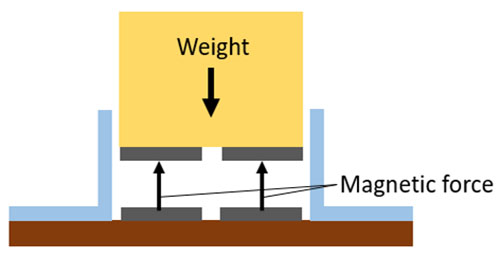Figure 1 – Magnetic Levitation

There are two types of magnetic levitation systems: Electromagnetic suspension (EMS) and electrodynamics suspension (EDS).  The Electromagnetic type uses the attractive force between the magnets, present on the bottom of the train, and the guideway, to lift the train. The magnets on the left and right sides of the train are used to eliminate any lateral displacement. This method is easier to implement and works even for zero speed.

In this application note, a proposed electromagnetic levitation system model in a semi-high-speed maglev train is analyzed using EMWorks2D. The proposed model consists of a type F rail, levitation electromagnets, and guidance electromagnets . The levitation magnets are used to lift off the vehicle from the ground to a specific position in the free space, while the guidance magnets are used to center the vehicle above the rail and eliminate any lateral displacement. The ferromagnetic components are made of steel 1010, while the windings are made of copper.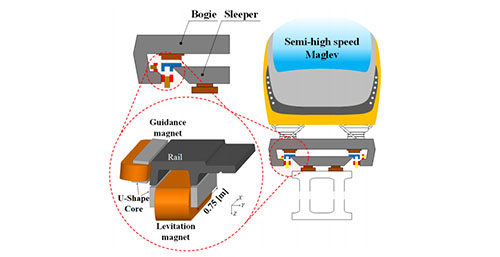Figure 2- The Electromagnetic Levitation System 

Figures 3a) and 3b) show respectively 3D and 2D CAD models built inside SOLIDWORKS. The 2D model is extracted from the 3D original geometry automatically using the 2D Simplification feature of EMWorks2D.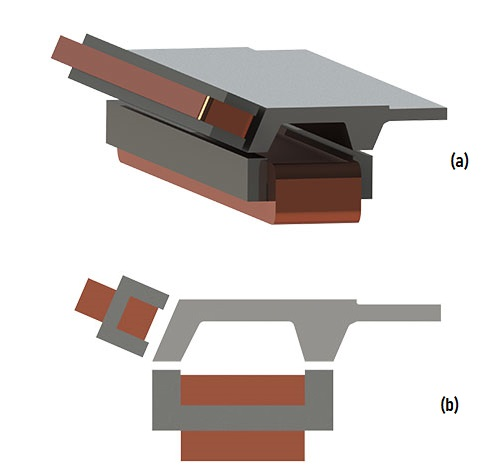Figure 3 - CAD Models of the Magnetic Levitation System, a) 3D Model, b) 2D Model 

## Simulation Results

Static magnetic simulations are performed using the Magnetostatic module of EMWorks2D. Parametric sweep feature is used to compute the magnetic field, levitation force, and guidance forces versus different parameters such as airgap length, applied current, etc. The levitation winding has 250 turns, while the guidance winding has 180 turns. The applied current is 15A and 7A respectively. Studying both levitation and guidance forces is particularly important to build an efficient and stable maglev system.

The figures below show the magnetic flux density plots several vertical airgap values. Clearly, the smaller is the airgap, the higher is the magnetic flux density. The flux results can be animated versus the parametric variable using EMWorks2D. Figure 6 contains an animation of the magnetic flux versus the Y position of the levitation magnet.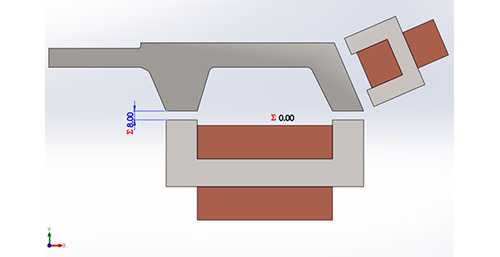Figure 4 - 2D Model With the Variable Airgap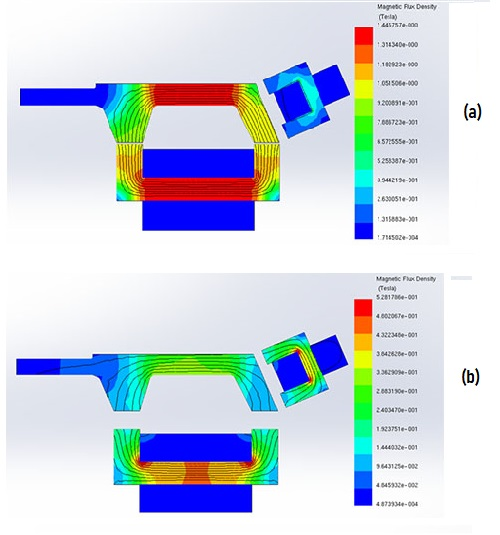Figure 5 - Magnetic Flux Results at Different Airgap Size, a) 2 mm, B) 20 mm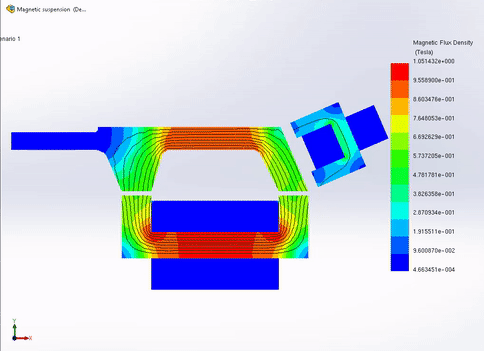Figure 6 - Animation of Magnetic Flux Density Versus Y Position

Figure 7 shows the force results versus the airgap size. The force decreases exponentially with the airgap. It has a peak value of 15 kN at 2 mm, while it is lower than 2 kN with an air gap smaller than 7 mm.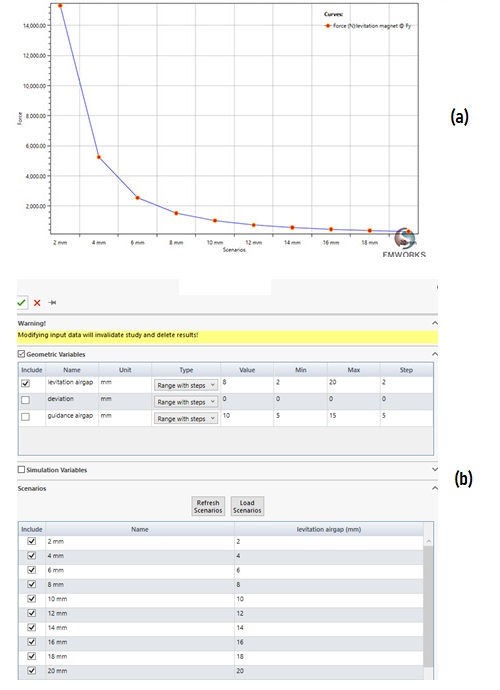Figure 7 - Force Results Versus Airgap Computed Using a Parametric Study of EMWorks2D, a) Force Curve, B) Design Scenarios Page of EMWorks2D.

Figure 8 contains a comparison between force results versus current at different airgap lengths; the higher is the current and the smaller is the airgap, the larger is the force.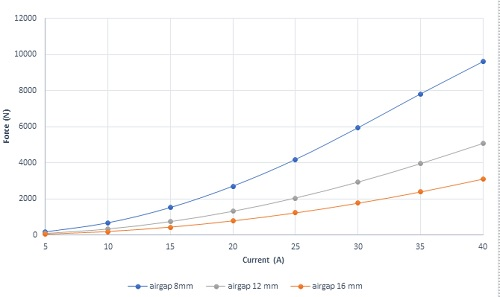Figure 8 - Force Results Versus Current at Different Airgap Distances

Figure 9 the lateral position of the rail is varied; both the levitation and guidance forces are measured. The lateral position of the rail impacts the stability of a maglev system. The rail should be maintained in an aligned position to maximize the levitation force and reduce the losses.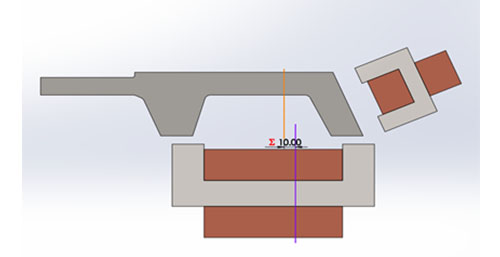Figure 9 - 2D Model With Lateral Deviation

Figures 10a) and 10b) show the magnetic flux results at a maximum lateral deviation for an airgap of 10 mm. Figure 10b contains a superimposed plot of the 2D field plot with a cross-section view of the 3D model.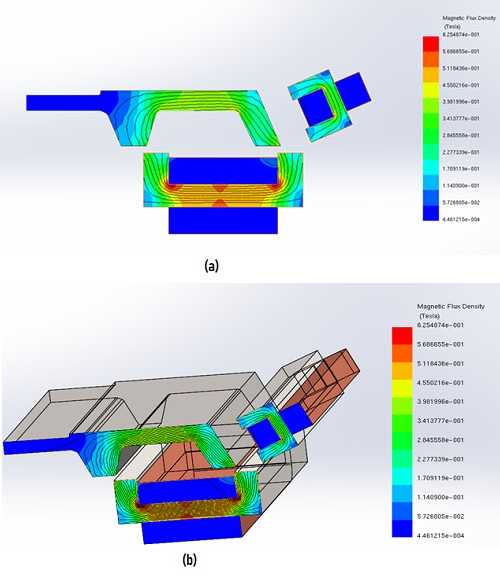Figure 10 - Magnetic Field Results, a) Plot on the 2D Model, b) Plot on the 3D Model

An animation of the magnetic field versus x position is shown in Figure 11; the airgap flux density decreases versus lateral displacement.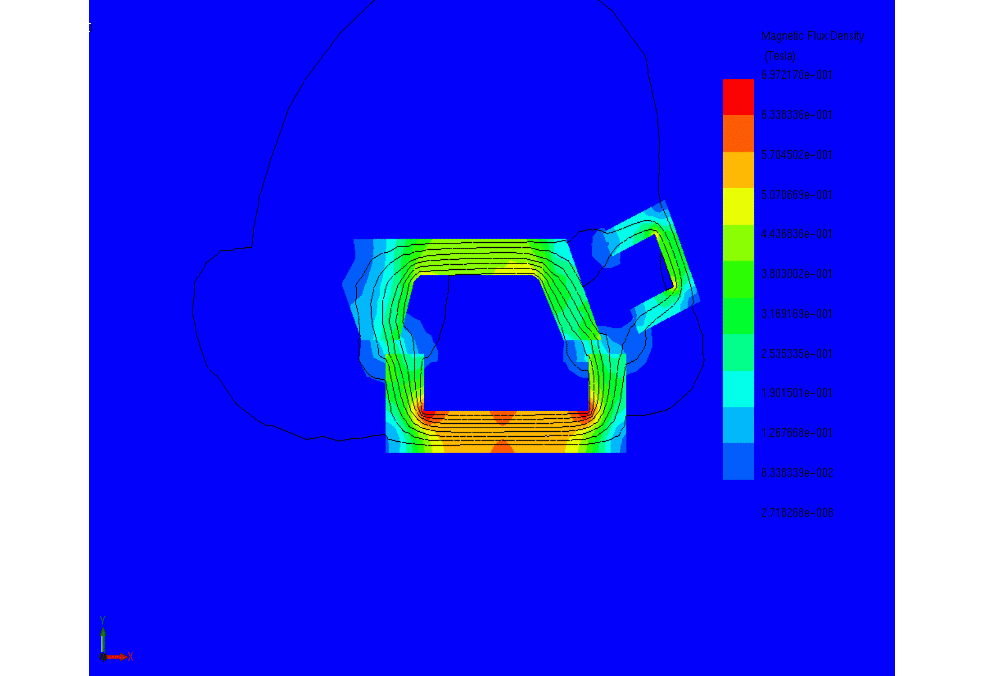Figure 11 - Animation Plot of the Magnetic Flux Density Versus the Lateral Deviation

Figures 12a) and 12b) show, respectively, the guidance and levitation forces versus deviation. The lateral force, which should be maintained at low values, increases with the lateral deviation. Unlike the lateral force, the levitation force decreases with the x position.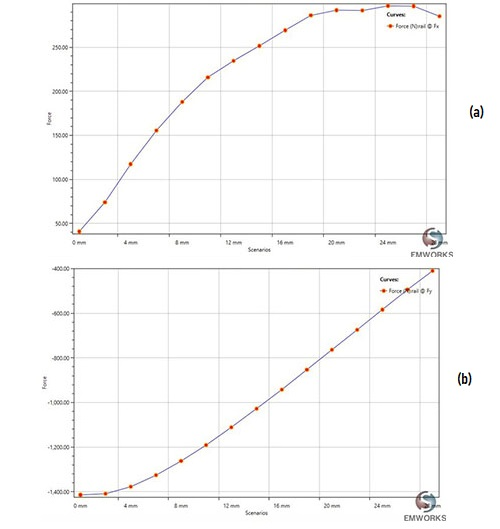Figure 12 - Magnetic Force Versus Lateral Deviation, a) Guidance Force, b) Levitation Force

The inductances, for both levitation and guidance coils, are shown in the Figure 13; the larger is the deviation in the x position, the smaller are the inductances.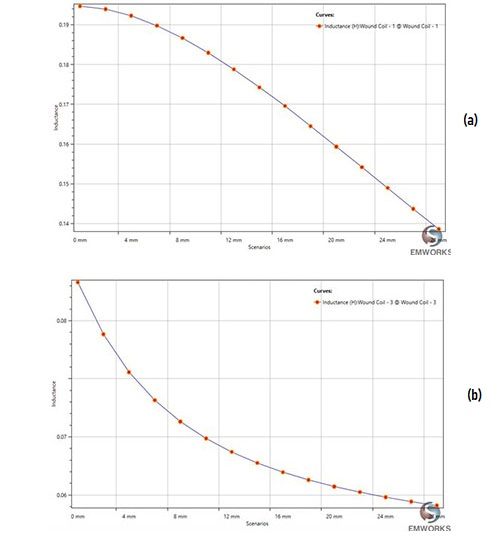Figure 13 - Inductances Results, a) Levitation Coil, b) Guidance Coil

## Optimizing the Levitation Force

Using the SolidWorks multi-configurations feature, the levitation force is computed for 3 different configurations; the results are shown below. The original configuration is shown in Figure 14a; a second configuration with two added magnets in lateral positions is shown in Figure 14b; a third configuration, with one added magnet at the bottom of the ferromagnetic core that has two coils instead of one coil, is shown in Figure 14c.  Figures 15a) and 15b) show SolidWorks multi-configuration page and EMWorks studies tree, respectively.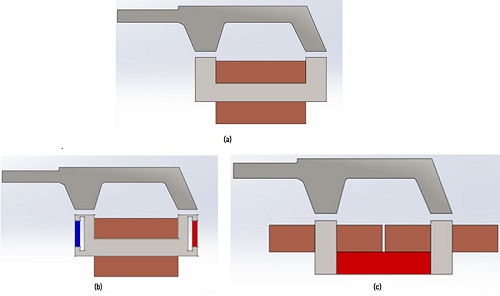Figure 14 - Simulated Configurations, a) Original Design, b) Design 2, c) Design 3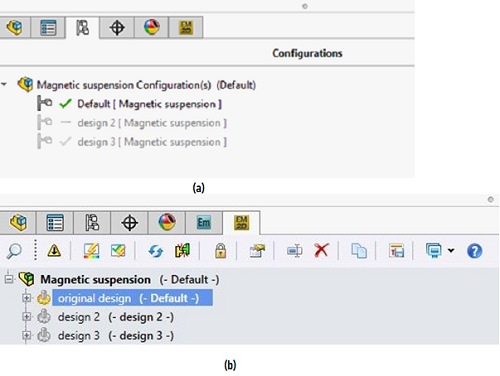Figure 15 - a) Multi-Configurations Feature Page, b) EMWorks2D Tree With Multi-Configurations

The magnetic field results for different configurations are plotted in Figure 16, while the force results are illustrated in Figure 17; the third configuration, with two adjacent coils, gives the highest force among the three configurations, which can be considered as an optimized system.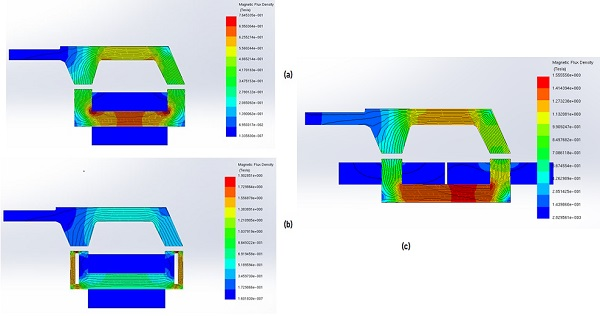Figure 16 - Magnetic Flux Results, a) Original Design, b) Design 2, c) Design 3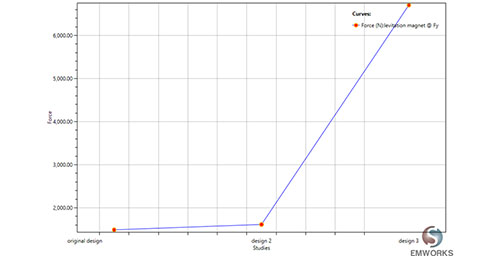Figure 17- Comparison of the Three Configurations in Terms of the Levitation Force.

## Conclusion

EMWorks2D is used to study and simulate an electromagnetic levitation system. A plethora of results are obtained including magnetic flux density, magnetic forces, and inductances; the airgap size plays an important role in the performance of the levitation system.

## Reference

: Min Kim, Jae-Hoon Jeong, Jaewon Lim†, Chang-Hyun Kim and Mooncheol Won. Design and Control of Levitation and Guidance Systems for a Semi-High-Speed Maglev Train. J Electr Eng Technol.2017; 12(1): 117-125